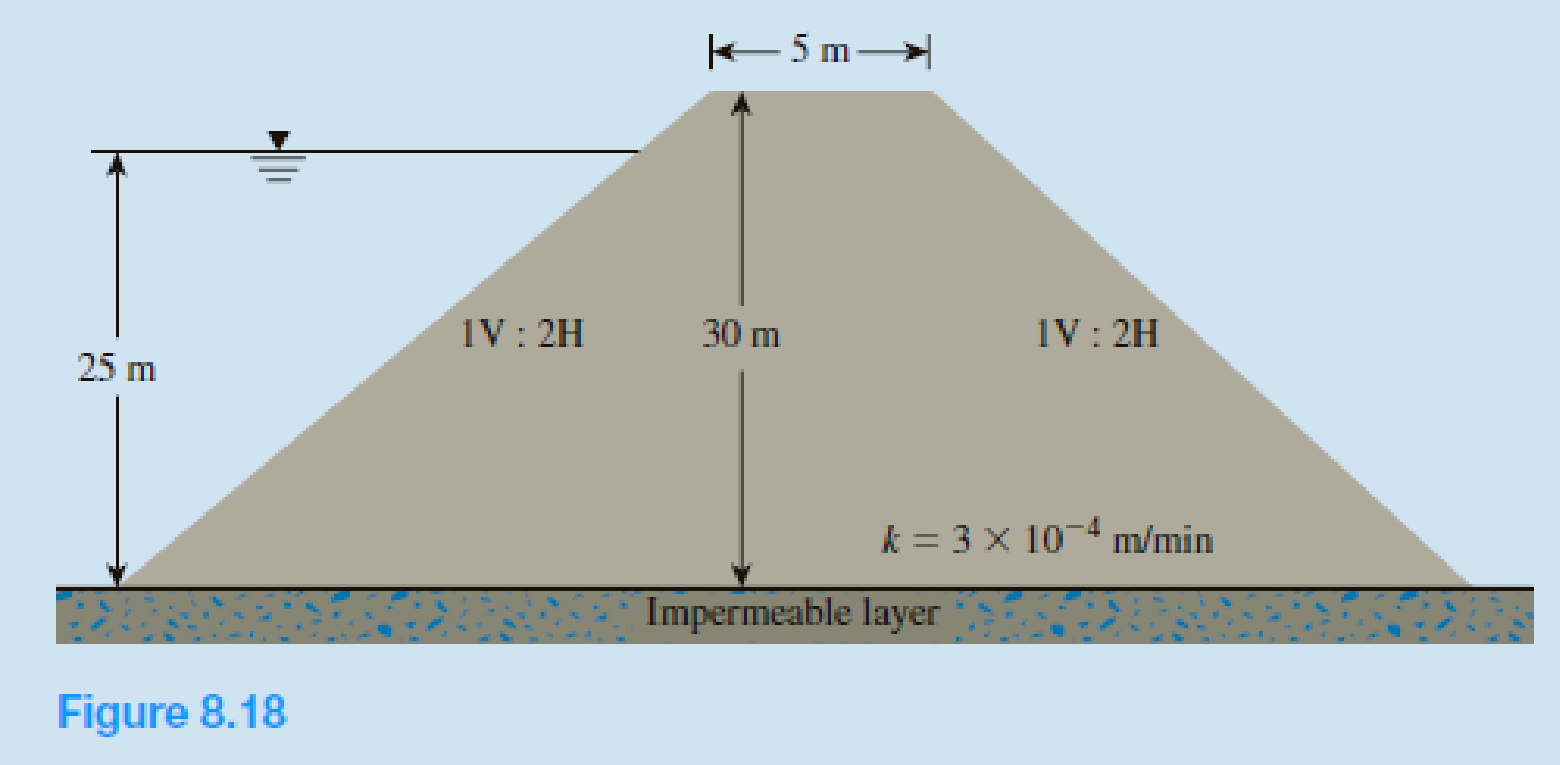Chapter 8, Problem 8.10PPrinciples of Geotechnical Enginee...

9th Edition
Braja M. Das + 1 other
ISBN: 9781305970939

Solutions

Chapter
SectionPrinciples of Geotechnical Enginee...

9th Edition
Braja M. Das + 1 other
ISBN: 9781305970939
Textbook Problem

Refer to the cross section of the earth dam shown in Figure 8.18. Calculate the rate of seepage through the dam (q in m3/min/m) using Schaffernak’s solution.To determine

Find the seepage rate through the dam q, in m3/min/m length using Schaffernak’s solution.

Explanation

Given information:

The height of the water level H is 25 m.

The height of the earth dam H1 is 30 m.

The length of earth dam top L1 is 5 m.

The hydraulic conductivity of the permeable soil layer k is 3×104m/min.

Calculation:

Refer Figure 8.18 in the textbook.

Determine the value of α using the relation.

tanα=VH

Here, V is the vertical distance in the slope and H is the horizontal distance in the slope.

Substitute 1 for V and 2 for H.

tanα=12α=tan1(0.5)α=26.57°

Determine the value of Δ using the relation.

Δ=2H

Substitute 25 m for H.

Δ=2×25=50m

Determine the distance d value using the relation.

d=2H1+L1+2(H1H)+0.3Δ

Substitute 30 m for H1, 5 m for L1, 25 m for H, and 50 m for Δ.

d=2×30+5+2(3025)+0.3×50=90m

Determine the length L value using the relation.

L=dcosαd2cos2αH2sin2α

Substitute 90 m for d, 26

Still sussing out bartleby?

Check out a sample textbook solution.

See a sample solution

The Solution to Your Study Problems

Bartleby provides explanations to thousands of textbook problems written by our experts, many with advanced degrees!

Get Started

What is game theory? Identify two applications of game theory.

Principles of Information Systems (MindTap Course List)

Explain the differences among data, information, and a database.

Database Systems: Design, Implementation, & Management

What is a relationship, and what three types of relationships exist?

Database Systems: Design, Implementation, & Management

Explain Appleâ€™s view of user interface design, especially for apps.

Systems Analysis and Design (Shelly Cashman Series) (MindTap Course List)

Determine the resultant of the force system shown.

International Edition---engineering Mechanics: Statics, 4th Edition